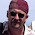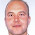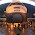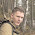Learning Clojure

Tuesday, August 20, 2013

Gibbs Sampler : Clojure vs Julia, Java, C, MATLAB, Scala and Python

I never could resist wading into someone else's fight. To be honest, I think Clojure and I lose this one pretty badly, even though it turns out to be easy enough to make it do the sampling at Javatic speeds.

;; Brother Jackson (http://boss-level.com) has recently been trying to
;; persuade me to use a new language.

;; He describes Julia (http://julialang.org) as being 'like Matlab,
;; but not crap', so it's clear that there are points both for and
;; against it.

;; As part of his campaign, he pointed me to
;; http://timsalimans.com/gibbs-sampling-with-julia/ and that lead to:
;; http://darrenjw.wordpress.com/2011/07/16/gibbs-sampler-in-various-languages-revisited/
;; which compares various languages on a simple gibbs sampler.

;; Darren's timings go from 7 minutes (for R) to 8 seconds (for C)
;; The point of Tim's posts is that Matlab *can* be persuaded to run this quickly,
;; and that Julia just does.

;; Two other languages that also do well are Java and Scala, with timings around
;; the 11 second mark for the simple Gibbs sampler benchmark.

;; This is a bit of a red rag to a bull, as far as I'm concerned. One
;; reason I like clojure so much is that it lets me think at a very
;; abstract level.

;; With most abstract languages, you pay a heavy speed penalty, and
;; Clojure's no exception, but generally, if it goes slowly enough to
;; annoy you, there's a simple way to speed it up.

;; So I'm thinking, 'If Java and Scala can go that quickly then surely
;; Clojure can'.  It's been a while since I last tried to speed
;; something up, and a lot has changed since.

;; Firstly, for comparison, I ran Darren's C program on my little
;; netbook and it took 91 seconds. The Java program took 147 seconds.
;; So I'm guessing his computer's about 11 times faster than mine,
;; give or take, but that the scale factor's about constant.

;; Here's the Java program that did rather well:

;; import java.util.*;
;; import cern.jet.random.tdouble.*;
;; import cern.jet.random.tdouble.engine.*;

;; class Gibbs
;; {

;;     public static void main(String[] arg)
;;     {
;;     int N=50000;
;;     int thin=1000;
;;     DoubleRandomEngine rngEngine=new DoubleMersenneTwister(new Date());
;;     Normal rngN=new Normal(0.0,1.0,rngEngine);
;;     Gamma rngG=new Gamma(1.0,1.0,rngEngine);
;;     double x=0;
;;     double y=0;
;;     System.out.println("Iter x y");
;;     for (int i=0;i<N;i++) {
;;         for (int j=0;j<thin;j++) {
;;         x=rngG.nextDouble(3.0,y*y+4);
;;         y=rngN.nextDouble(1.0/(x+1),1.0/Math.sqrt(2*x+2));
;;         }
;;         System.out.println(i+" "+x+" "+y);
;;     }
;;     }

;; }

;; The first thing to do is to translate this program into clojure.

;; First we want to add the Parallel Colt library on which this
;; program depends Since restarting the repl is pretty much a fate
;; worse than death we can use the admirable pomegranate library
(require 'cemerick.pomegranate)
:coordinates '[[net.sourceforge.parallelcolt/parallelcolt "0.10.0"]])

;; Using Parallel Colt was a bit of a pain in java, requiring fiddling
;; with jars and classpaths and poms.xml, and the corresponding libgsl
;; for the C program required a weird bit of debugging before I could
;; get it to work. But the combination of pomegranate and maven
;; makes adding libraries child's play.

;; On the other hand, you do have to import every java class that you
;; intend to use in Clojure by hand. There's no way to translate * as
;; far as I know.
(import (cern.jet.random.tdouble.engine DoubleMersenneTwister))
(import (cern.jet.random.tdouble Normal Gamma))
(import java.util.Date)

;; If we care about speed in Clojure, then this is always a cool thing to do:
(set! *warn-on-reflection* true)
;; The point of it is to warn you when Clojure can't find the type of
;; a java object at compile time.  When this happens, it uses
;; reflection, and if that happens in a tight loop, it can be
;; *extremely* slow

;; So, here we go:

(def N 50000)
(def thin 1000)

(def rngEngine (DoubleMersenneTwister. (Date.)))

(def rngN (Normal. 0.0,1.0,rngEngine))
(def rngG (Gamma.  1.0,1.0,rngEngine))

;; I'm translating as literally as possible here, but I'm going to
;; leave the printing out until the end so that I can play with the
;; program without generating screenfuls of crap.

;; The original is very imperative, and doesn't really fit
;; Clojure's natural style. You can't just change the values of things
;; in Clojure, you have to use Software Transactional Memory.

;; This has good and bad aspects, but when you're trying to make a
;; literal translation of an imperative program it looks very ugly,
;; and it's going to have a huge overhead in a calculation like this.

(defn literal[]
(let [x (atom 0) y (atom 0)]
(dotimes [i N]
(when (zero? (mod i 10)) (println i)) ;; Printing a progress counter
(dotimes [j thin]
(swap! x (fn[_] (.nextDouble rngG 3.0 (+ (* @y @y) 4))))
(swap! y (fn[_] (.nextDouble rngN (/ (+ 1 @x)) (/ 1.0 (Math/sqrt (+ 2 (* 2 @x)))))))))))

;; Notice that as soon as you define this function, you get:
;; Reflection warning, NO_SOURCE_PATH:6 - call to nextDouble can't be resolved.
;; Reflection warning, NO_SOURCE_PATH:7 - call to nextDouble can't be resolved.

;; And the performance is truly appalling! There is no way I am ever
;; going to wait for this to finish, but I calculate that it will take
;; around an hour and a half. That's quite a slowdown, and it puts
;; Clojure in the same category as R and (badly written) MATLAB

(time (literal)) ;-> let's say about 90 minutes or so.

;; Luckily, reflection warnings are easy to fix.
;; You just tell the compiler the type of the mysterious object:
(defn literal-with-reflections-fixed[]
(let [x (atom 0) y (atom 0)]
(dotimes [i N]
(when (zero? (mod i 10)) (println i))
(dotimes [j thin]
(swap! x (fn[_] (.nextDouble ^Gamma rngG 3.0 (+ (* @y @y) 4))))
(swap! y (fn[_] (.nextDouble ^Normal rngN (/ (+ 1 @x)) (/ 1.0 (Math/sqrt (+ 2 (* 2 @x)))))))))))

;; That gives us a gigantic speedup, but the performance is still poor
(time (literal-with-reflections-fixed)) ;-> about 9 minutes

;; Remembering that Java took 2 1/2 minutes, we have a factor of four
;; to get rid of here.  That's actually much better than I was
;; expecting given this awful abuse of STM. I'll be less wary of using
;; it heavily in future.

;; But the obvious bottleneck is still all that use of the STM
;; mechanism in the middle of the tight inner loop.  Besides, it's
;; just nasty. That's really not what Clojure's heavily guarded
;; mutable state is for.

;; Let's get rid of that and replace it with clojure's natural loop/recur mechanism
;; in the inner loop, where we're just throwing away the intermediate values,
;;  and use the elegant iterate for the outer loop, where we want them.

(defn gibbs-loop [[x y]]
(loop [j thin x x y y]
(let [x (.nextDouble ^Gamma rngG 3.0 (+ (* y y) 4))
y (.nextDouble ^Normal rngN (/ (+ 1 x)) (/ 1.0 (Math/sqrt (+ 2 (* 2 x)))))]
(if (> j 0) (recur (dec j) x y )
[x y]))))

(def samples (iterate gibbs-loop [0 0]))

;; Now, how long does it take us to get our 50000th sample?
(time (nth samples N))
;; 219 seconds. (vs 147 for Java/Scala and 91 seconds for C )

;; And I think at that point I'm happy.

;; It's probably possible to bum a few more cycles out of this code, but it's hard to think of why you'd care.

;; Clojure's never going to run faster than Java when you're asking
;; them to perform the same computation, So the best we could hope for
;; is to get rid of the 50% slowdown over Java. I'm sure that's
;; possible, but I think the code above is a nice compromise between
;; speed, elegant expression of the idea, and flexibility. All of
;; which are important in real programs.

;; I did say that I'd cover writing all this to a file as well (and numbering the iterations), so

(time (spit "delete.me" (apply str (map (fn [i [x y]] (str i " " x " " y "\n")) (range) (take N samples)))))
;; 3 seconds. Told you that wasn't the hard part.

;; What I really have no idea how to do, let alone to do fast, is to
;; make a traditional unix-style command line utility that can be run
;; from the command prompt and write to stdout.

;; I imagine it's possible, but the amount of
;; library/classpath/maven/#! trickery required is going to be
;; immense. And I don't think there's any getting away from the fact
;; that clojure takes ages to start up.

;; That's a real shame, because I really love that style of programming. I miss it.

;; I wish that there was a language like clojure that lived in the traditional UNIX/C world rather than on the JVM.

;; Pretty much everything about the JVM drives me up the wall. But
;; actually it's not the JVM's fault that clojure takes ages to
;; start. The Java version of this program starts almost instantly.

;;;;;;;;;;;;;;;;;;;;;;;;;;;;;;;;;;;;;;;;;;;;;;;;;;;;;;;;;;;;;;;;;;;;;;;;;;;;;;;;;;;;;;;;;;;;;;;;;;;;;;;;;;;;;;;

;; Footnotes

;; If you'd written this sampler in clojure in the first place, your
;; original code would probably have looked something like this:

(defn gibbs [[x y]]
(let [x (.nextDouble rngG 3.0 (+ (* y y) 4))
y (.nextDouble rngN (/ (+ 1 x)) (/ 1.0 (Math/sqrt (+ 2 (* 2 x)))))]
[x y]))

(defn chain [thin [x y]]
(nth (iterate gibbs [x y]) thin))

(def samples  (iterate (partial chain thin) [0 0]))

;; Notice the reflection warnings!

;; If you then fix the reflections:

(defn gibbs [[x y]]
(let [x (.nextDouble ^Gamma  rngG 3.0 (+ (* y y) 4))
y (.nextDouble ^Normal rngN (/ (+ 1 x)) (/ 1.0 (Math/sqrt (+ 2 (* 2 x)))))]
[x y]))

(defn chain [thin [x y]]
(nth (iterate gibbs [x y]) thin))

(def samples  (iterate (partial chain thin) [0 0]))

;; Then you get code which isn't too shabby.

(time (nth (iterate (partial chain thin) [0 0]) 100))
;; 540msec

(time (nth (iterate (partial chain thin) [0 0]) 1000))
;; 5542msec

(time (nth (iterate (partial chain thin) [0 0]) 10000))
;; 56353 mseconds

(time (nth (iterate (partial chain thin) [0 0]) 50000))
;; 319 seconds. About 1/2 the speed of Java.

;; But you end up spending about half the program time packing and
;; unpacking [x y] pairs and creating and garbage collecting a lazy
;; seq that you're never going to look at.

;; I think it's worth having the explicit loop/recur in the inner loop

;; On the other hand:

(defn samples-loop [N]
(loop [x 0.0 y 0.0 j thin i N acc []]
(let [x (.nextDouble ^Gamma rngG 3 (+ (* y y) 4))
y (.nextDouble ^Normal rngN (/ (+ 1 x)) (/ 1 (Math/sqrt (+ 2 (* 2 x)))))]
(if (> j 0) (recur x y (dec j) i acc)
(if (> i 0) (recur x y thin (dec i) (conj acc [x y])))))))

;; Has clearly left the path of righteousness.

;; Although it does achieve the speed for which it sold its soul.

(time (last (samples-loop 50000)))
;; 182 seconds

1.Brilliant as always, John. Kudos.

2.Instead of parallelcolt you can look to Clatrix that wraps the JBlas...

3.I get to be the baddie - love it.

On my laptop (time (last (samples-loop 50000))) takes ~1750msec.

Lifting and updating from http://dmbates.blogspot.co.uk/2012/05/simple-gibbs-example-in-julia.html here's the equivalent code Julia. Ie no funny tricks with parallelism

------------------
using Distributions
function JGibbs(N::Int, thin::Int)
mat = Array(Float64, (N, 2))
x = 0.
y = 0.
for i = 1:N
for j = 1:thin
x = rand(Gamma(3)) * (y*y + 4)
y = 1/(x + 1) + randn()/sqrt(2(x + 1))
end
mat[i,:] = [x,y]
end
mat
end

@elapsed JGibbs(50000, 200)
------------------------------

That takes 680msec, which is hard to argue with.

Brother Jackson

1.Is your laptop really 90x faster than my netbook? What the hell? I thought Moore's Law had stopped?

2.You probably timed Julia's start up time too. It's pretty funny how Julia is hyped for performance but the start-up is so slow.

4.For comparisons like this why not seed the RNG with the same constant every time.

1.Probably should, but there's not a great deal of noise in the signal from that. It would also be a good idea to force garbage collection and give the code enough runs through that the JIT has time to optimize it, both of which are noticeable effects. And there are bound to be other factors.

But to be honest, I don't really care about anything but the order-of-magnitude! Looks like I can get two of those by stealing Ed's laptop.

2.Its so heavy to carry you'd never make good your getaway :)

3.Indeed, it might sink your boat !

5.You can squeeze a little bit more performance with following:
-(set! *unchecked-math* true)
-ensure that "thin" and "N" are casted to longs instead of being Objects. In your last example they are Objects;
-introduce type Pair instead of constructing a vector every time.
This gives an additional 5-10% in my tests.

6.There is also an additional quirk if you use leiningen: https://github.com/technomancy/leiningen/wiki/Faster#tiered-compilation By default, leiningen use some JVM options that hurt performance.

7.Bit late to this. Once the clojure loops are written imperatively using pre-allocated double arrays, the clojure speed is about the same as Java and in my tests takes about 45% longer than the code posted by Edmund (who pre-allocates the array). The following library has some good Java PRNGs http://www.iro.umontreal.ca/~simardr/ssj/indexe.html.. I timed the following code taking 45% longer than the Julia:
(import (umontreal.iro.lecuyer.rng MT19937 MRG32k3a))
(import (umontreal.iro.lecuyer.randvar NormalGen GammaAcceptanceRejectionGen ))
(def SSJrngEngine (MT19937. (MRG32k3a.)))
(defn samples-loop [^long N ^long thin]
(loop [x 0.0 y 0.0 j thin i N acc (make-array Double/TYPE N 2)]
(let [x (*(GammaAcceptanceRejectionGen/nextDouble SSJrngEngine 3.0 1.0 )(+ (* y y) 4.0))
y (+ (/(NormalGen/nextDouble SSJrngEngine 0.0 1.0)(Math/sqrt (+ 2.0 (* 2.0 x)))) (/ 1.0 (+ 1.0 x))) ]
(if (> j 0) (recur x y (dec j) i acc)
(if (> i 0) (do
(let [^doubles dacc (aget ^objects acc (- N i))]
(aset dacc 0 x )
(aset dacc 1 y )
)
(recur x y thin (dec i) acc)))))))

Since Gamma PRNGs call Gaussian and Uniform PRNGs, this story largely reflects the speed of pure Java vs C uniform and normal PRNGs (calling the PRNGs dominate the numerical loops). The expensive parts of the Julia Uniform and Gaussian PRNGs are coded in c: https://github.com/JuliaLang/Rmath/blob/master/src/randmtzig.c. Not surprising that pure java version take about 45% longer which is roughly the original Java vs C comparison. There are also algorithmic differences between e.g. Julia's C Gaussian PRNG and the various Java ones. This one is well regarded in finance: http://home.online.no/~pjacklam/notes/invnorm/#Java

A multivariate Gaussian comparison would be interesting given that Clojure matrices are a recent development but Julia has a matrix focus.